Elite indicators :) - page 4532970
Hercs

Regression Channel

Mladen, I downloaded a "Regression Channel.mq4" from you and sadly I did not put the reference Thread number to it. (I found the referenced Thread No. @ Page 408 #4072) This one I like the fact that one can select Hi/Lo too, but would there be any sense to make it Tr.Env. type? I guess not as we need to see when the price goes beyond the Line, right?

What I need to know please, is whether the "Calculating Mode" (set to 0 ) should be read as 0 = SMA and 1 = EMA, etc. or is this what you meant by saying that it is sometimes called LSMA?

I read an interesting article by Richard L. Muehlberg on trading the Regression Channels and he mentions using an 11-day period on one of his charts and 9 on another and both showed absolute "indications" of when to trade. Is it possible to get such an addition or not? You may have information that could negate the need for settings such as those mentioned above in which case I would like to hear about them, plse.

I look forward to hearing from you.

Best regards.151619

Regression channel ...

ValeoFX

In regression channel, calculating mode refers to what prices will it use in calculation and what kind of a channel it will make.

mode 0 -> uses the "classical" calculation : close for regression line and close is used for deviations calculation

mode 1 -> uses high for one regression line and low for another regression line. Deviations are also calculated using high and low

mode 2 -> uses close for regression line and high and low for deviations.

modes 1 and 2 are asymmetrical : upper part of the channel is calculated using high price and lower is calculated low price so they do not (and almost never are) have to be the same (in distance from regression line) at all. Frankly, I like the mode 1 the best (that chart is one of the usual ones I have : regardless of the recalculating nature of regression channel, I find it helpful in my trading)

As of days : here is a version that will translate the number of days you want to use into bars (set the daysCalculation to true and set the daysNumber to desired number of days). It will work that way for time frames less than or equal to daily time frame. For weekly and monthly charts it will use the bars parameter instead regardless of the daysCalculation setting). Here is an 11 day example of a regression channel (mode 1 : highs and lows for all)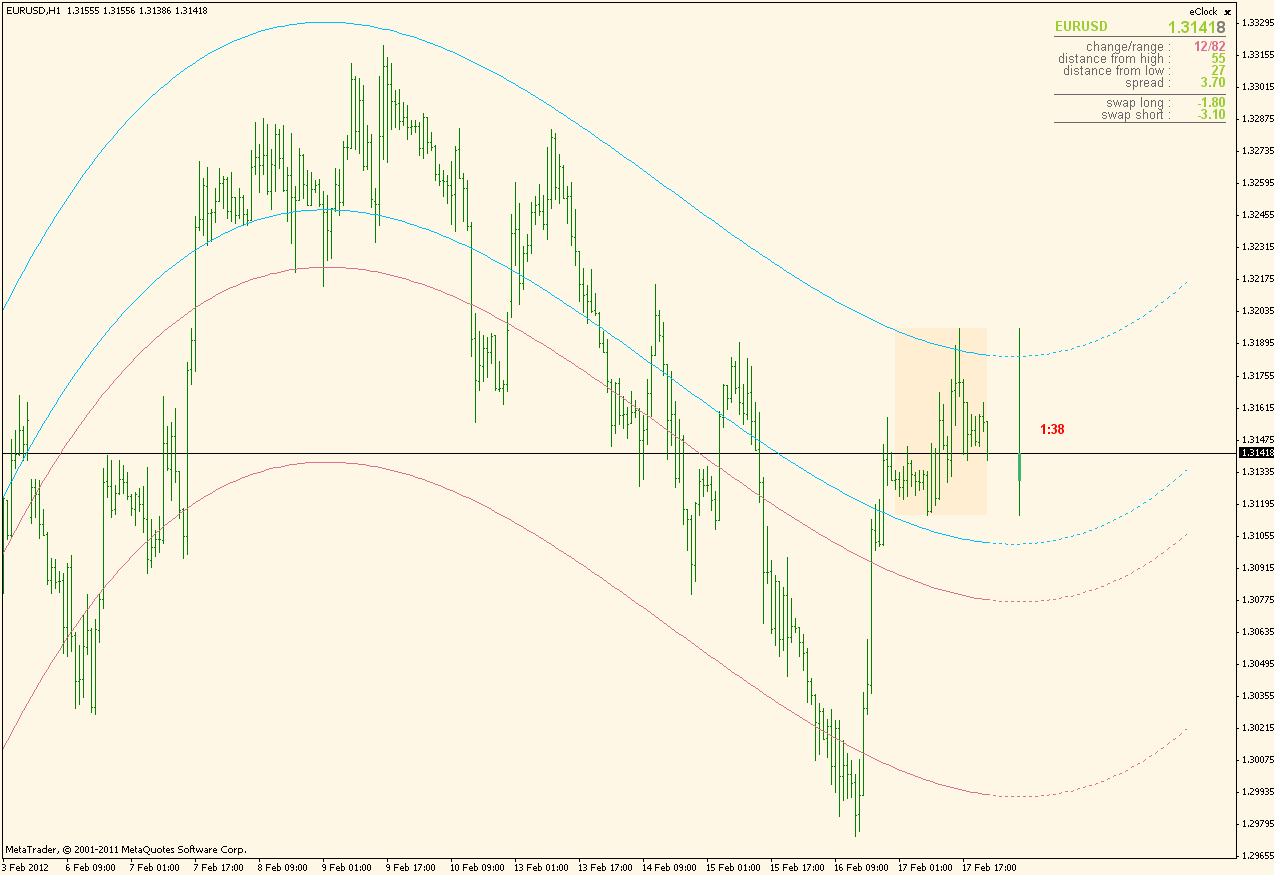Update version posted here : https://www.mql5.com/en/forum/general

ValeoFX:
Mladen, I downloaded a "Regression Channel.mq4" from you and sadly I did not put the reference Thraed number to it. Only reminder is 8 September and I will have to start hunting it down round that time, probably more than a year ago now.

What I need to know please, is whether the "Calculating Mode" (set to 0 ) should be read as 0 = SMA and 1 = EMA, etc.

I read an interesting article by Richard L. Muehlberg on trading the Regression Channels and he mentions using an 11-day period on one of his charts and 9 on another and both showed absolute "indications" of when to trade. Is it possible to get such an addition or not? You may have information that could negate the need for settings such as those mentioned above in which case I would like to hear about them, plse.

I look forward to hearing from you.

Best regards.
Files:2970
Hercs

ValeoFX

In regression channel, calculating mode refers to what prices will it use in calculation and what kind of a channel it will make.

mode 0 -> uses the "classical" calculation : close for regression line and close is used for deviations calculation

mode 1 -> uses high for one regression line and low for another regression line. Deviations are also calculated using high and low

mode 2 -> uses close for regression line and high and low for deviations.

modes 1 and 2 are asymmetrical : upper part of the channel is calculated using high price and lower is calculated low price so they do not (and almost never are) have to be the same (in distance from regression line) at all. Frankly, I like the mode 1 the best (that chart is one of the usual ones I have : regardless of the recalculating nature of regression channel, I find it helpful in my trading)

As of days : here is a version that will translate the number of days you want to use into bars (set the daysCalculation to true and set the daysNumber to desired number of days). It will work that way for time frames less than or equal to daily time frame. For weekly and monthly charts it will use the bars parameter instead regardless of the daysCalculation setting). Here is an 11 day example of a regression channel (mode 1 : highs and lows for all)====================

Hey "youngman" you are suppose to have a quiet weekend?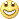Thank you so much for the explanation; as always precise and to the point. Much appreciated.

Also for the indicator; deeply indebted to you.

Best regards.2970
Hercs

Stochastic Volatility

I have not had time to evaluate your "Stochastic Volatility" indicator, as much as I like both concepts and so today I was looking at it. I show you a picture here for reference.

What surprises me is that it does not show the Dots in the TOP section in o/bought? Is there an improved version perhaps or is it meant to be like this? I can hardly believe me asking this question.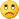Files:23

Wilder's dmi alert and EA request

Hello gentleman. I thank you for your efforts in this forum as it has helped me immensely. I would like ask for an alert with arrows when the DMI line crosses up over the ADX line. This is usually seen with a trend that has a high likelihood of continuatuion. If you all would be so kind to make it into an EA as well that would be wonderful. The exit for the system is for a percentage upon the DMI crossing back under the ADX and then the remainder when the ADX turns back down. Alerts could also accompany the exit signals of course. I have not seen any indicators or EAs that do this exactly anywhere. Thanks for your efforts in this regard.

Files:151619

...

Here is this one

I used the one that is coded to the last letter how Welles Wilder described it. Value differences between this one and the one you posted are marginal though so you will not notice any significant change in values.

Alerts added and can be configured separately : do you wish to be alerted when DMI+ or DMI- crosses ADX up or down separately (default is that it alerts only when DMI lines cross ADX up, but you can turn the alerts for down crosses on too, or you can turn just the down crosses on - it depends what exactly do you want to be alerted on)

Updated version posted here : https://www.mql5.com/en/forum/general

eric gellasch:
Hello gentleman. I thank you for your efforts in this forum as it has helped me immensely. I would like ask for an alert with arrows when the DMI line crosses up over the ADX line. This is usually seen with a trend that has a high likelihood of continuatuion. If you all would be so kind to make it into an EA as well that would be wonderful. The exit for the system is for a percentage upon the DMI crossing back under the ADX and then the remainder when the ADX turns back down. Alerts could also accompany the exit signals of course. I have not seen any indicators or EAs that do this exactly anywhere. Thanks for your efforts in this regard.
Files:154

Enclosed I have a Schaff Trend cycle indicator in histogram form. I have 2 wishes:

1) Please add msg alert when colors change from red to green and green to red eg: STC "BUY"EURUSD at 1.2850 or STC "SELL" EURUSD at 1.2836

2)There is a solid silver line that follows the histo...could it change along with the colored bars?

I have both the live version & the histo version. Presently I overlay one on top of the other; would like it combined if possible

Many thanks in advancestc_color-hiso.mq4

Files:151619

Composite RSI 2 ...

Updated and upgraded composite RSI.

Code is revised and as a result it is much smaller code now and allows calculations of up to depth 25. Here is an example of a 10 period and depth 10 calculation :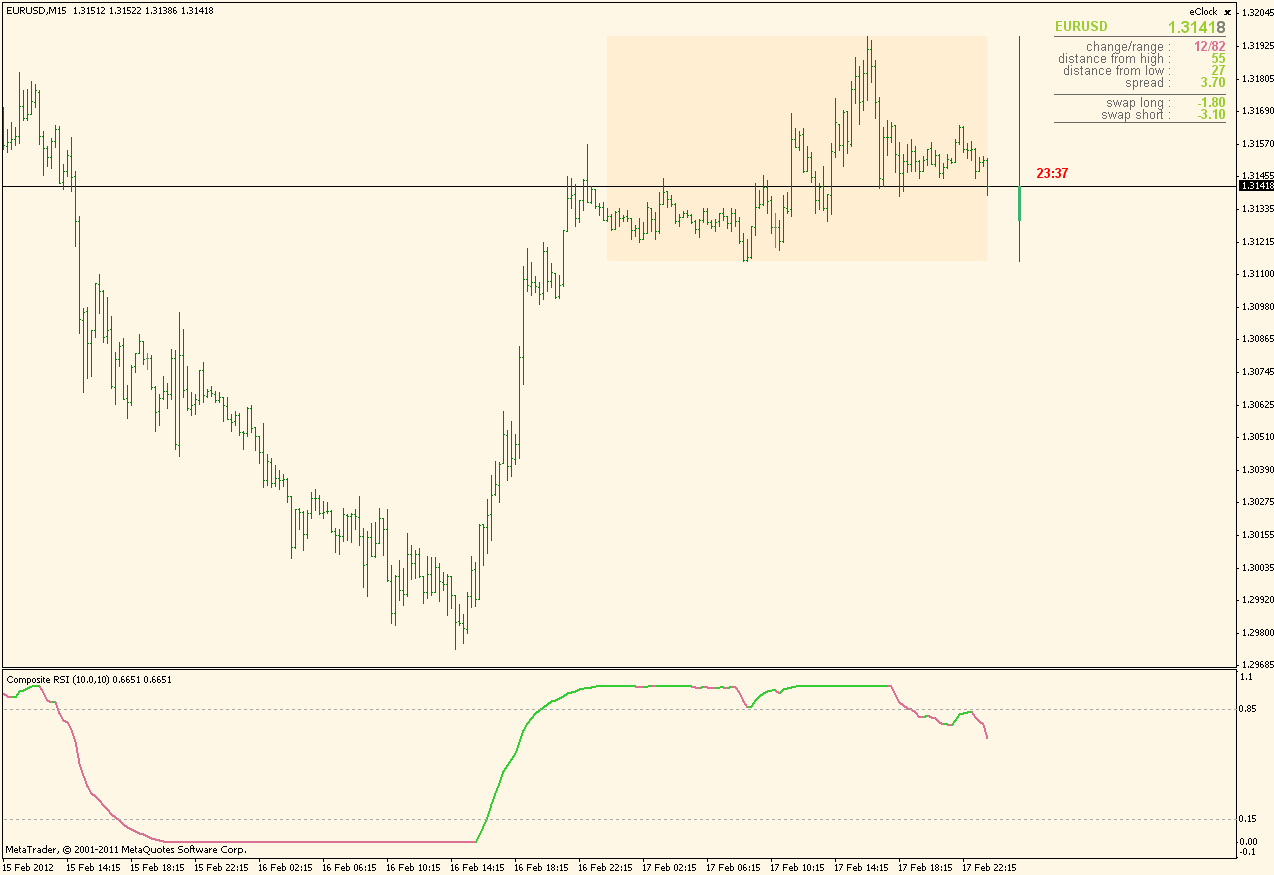Updated version posted here : https://www.mql5.com/en/forum/general

Files:151619

Heiken ashi smoothed with averages used in (the 18types of averages). Since price smoothing and result smoothing types can be different, combining the 18 types of averages gives us a very large number of possible Heiken ashi types this indicator "covers"

Averages types that can be used in it :
0 = SMA

1 = EMA

2 = Double smoothed EMA

3 = Double EMA (DEMA)

4 = Triple EMA (TEMA)

5 = Leader EMA

6 = Smoothed MA

7 = Linear weighted MA

8 = Parabolic weighted MA

9 = Alexander MA

10 = Volume weighted MA

11 = Hull MA

12 = Triangular MA

13 = Sine weighted MA

14 = Linear regression

15 = IE/2

16 = Non Lag MA

17 = Zero lag EMA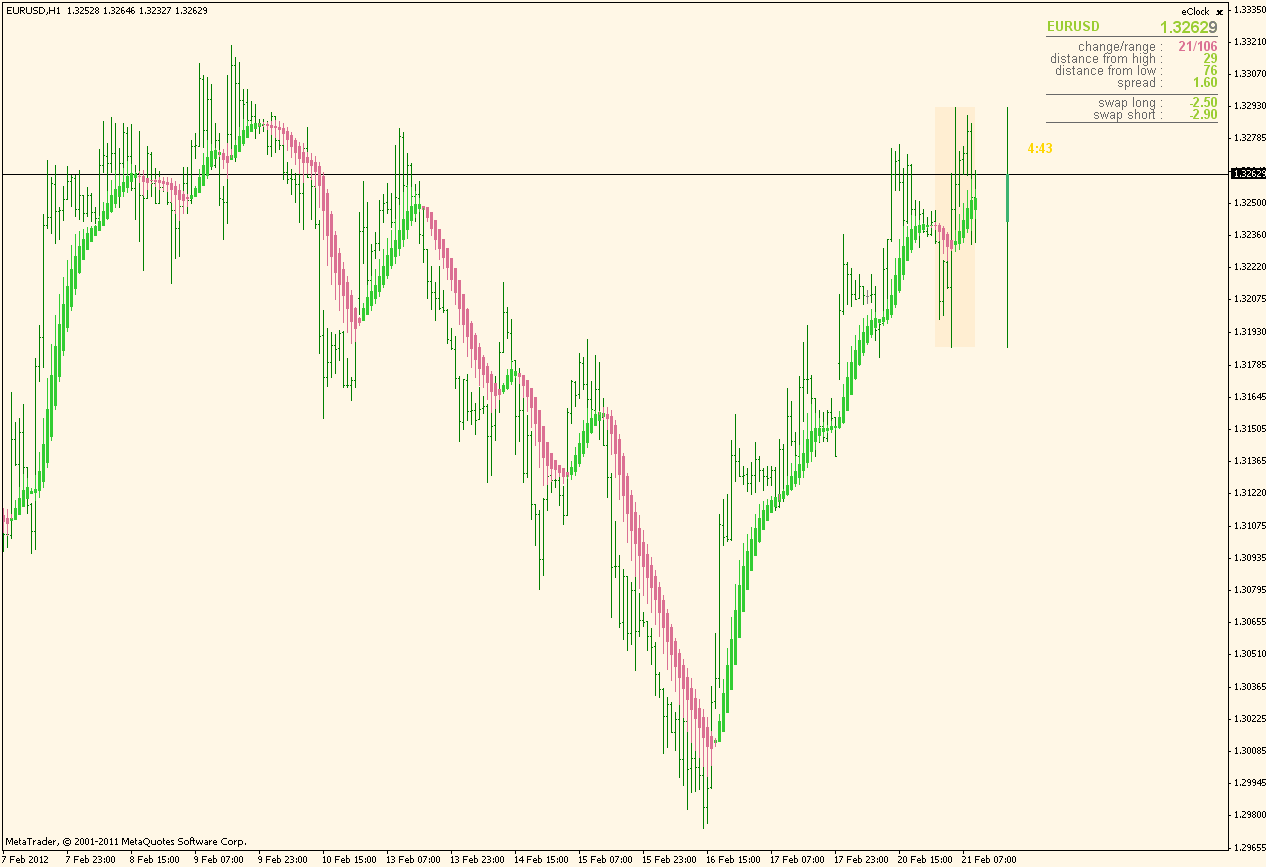Files:2970
Hercs

Explanation plse..

Heiken ashi smoothed with averages used in (the 18types of averages). Since price smoothing and result smoothing types can be different, combining the 18 types of averages gives us a very large number of possible Heiken ashi types this indicator "covers" Averages types that can be used in it :
0 = SMA

1 = EMA

2 = Double smoothed EMA

3 = Double EMA (DEMA)

4 = Triple EMA (TEMA)

5 = Leader EMA

6 = Smoothed MA

7 = Linear weighted MA

8 = Parabolic weighted MA

9 = Alexander MA

10 = Volume weighted MA

11 = Hull MA

12 = Triangular MA

13 = Sine weighted MA

14 = Linear regression

15 = IE/2

16 = Non Lag MA

17 = Zero lag EMA============

Looks impressive to say the least, but I need some explanation plse.

What is meant by the #1 line PriceSmoothing? and can I set that to any of the values stipulated?

#2 Line is PriceSmoothingMethod = is this the only one reserved for all the different methods?

#3. ResultSmoothing is a new concept to me in its entirety. Can I also use any of the 17 methods?

#4. ResultSmoothingMethod as for #2?

Would appreciate an explanation.

Thanking you in advance.